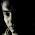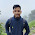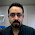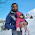Line Detection by Hough Line Transform

void HoughLines(InputArray image, OutputArray lines, double rho, double theta, int threshold, double srn=0, double stn=0 )
Parameters:
• image – 8-bit, single-channel binary source image (use edge detectors)
• lines – Output vector of lines. Each line is represented by a two-element vector.is the distance from the coordinate origin(top-left corner of the image).is the line rotation angle in radians ().
• rho – Distance resolution of the accumulator in pixels.
• theta – Angle resolution of the accumulator in radians.
• threshold – Accumulator threshold parameter. Only those lines are returned that get enough votes (>threshold ).
• srn – For the multi-scale Hough transform, it is a divisor for the distance resolution rho . The coarse accumulator distance resolution is rho and the accurate accumulator resolution is rho/srn . If both srn=0 and stn=0 , the classical Hough transform is used. Otherwise, both these parameters should be positive.
• stn – For the multi-scale Hough transform, it is a divisor for the distance resolution theta.
A good example for Hough Line Transform is provided in OpenCV Documentation.

Steps:

1. Load image and convert to gray-scale.
2. Apply the Hough Transform to find the lines.(HoughLines)
3. Draw the detected lines.(line)
4. Show the result

Example:

-------------
#include "opencv2/highgui/highgui.hpp"
#include "opencv2/imgproc/imgproc.hpp"
#include <iostream>

using namespace cv;
using namespace std;

int main()
{
Mat src = imread("building.jpg", 0);

Mat dst, cdst;
Canny(src, dst, 50, 200, 3);
cvtColor(dst, cdst, CV_GRAY2BGR);

vector<Vec2f> lines;
// detect lines
HoughLines(dst, lines, 1, CV_PI/180, 150, 0, 0 );

// draw lines
for( size_t i = 0; i < lines.size(); i++ )
{
float rho = lines[i], theta = lines[i];
Point pt1, pt2;
double a = cos(theta), b = sin(theta);
double x0 = a*rho, y0 = b*rho;
pt1.x = cvRound(x0 + 1000*(-b));
pt1.y = cvRound(y0 + 1000*(a));
pt2.x = cvRound(x0 - 1000*(-b));
pt2.y = cvRound(y0 - 1000*(a));
line( cdst, pt1, pt2, Scalar(0,0,255), 3, CV_AA);
}

imshow("source", src);
imshow("detected lines", cdst);

waitKey();
return 0;
}

-------------

Extra Stuffs:

• What if you need to select some lines on the basis of prior knowledge of range of angles?
So, you know the range of angles in which your lines may present. Now, what you need to do is to add a conditional statement to filter out the lines detected in that angle range. For example,

if you want to detect vertical lines, use the following conditional statement after line 23 of the above example.
if( theta>CV_PI/180*170 || theta<CV_PI/180*10)
{ Point pt1, pt2;
..........
line( cdst, pt1, pt2, Scalar(0,0,255), 3, CV_AA);
}

if you want to detect horizontal lines, use
if( theta>CV_PI/180*80 && theta<CV_PI/180*100)
{ Point pt1, pt2;
..........
line( cdst, pt1, pt2, Scalar(0,0,255), 3, CV_AA);
}

1.If i know that the lines im trying to detect will be in a specific range, e.g between 80 and 110 degrees, can I limit the range that houghLines searches through?

1.Excellent question Borg.

I have updated the post. Please have a look.
All you need to do is restrict your angle range. i.e. choose the lines those fall in your angle range. This can be done using a conditional statement after line detection.

2.Hi Kanha,
Hope you can teach on the specific degree. What I had understand is totally weird

?

1
2
3
4
5

if( theta>CV_PI/180*170 || theta 170 degree || means OR in C++ .. and still confusing.. please help to details out then.. appreciate on yr response
zamani

3.Here "theta" is in radian. So we have to convert degree to radian.
Here 170 degree = (CV_PI/180)*170 radian.

We may not get perfect horizontal lines. So we have to put some threshold. Perfect horizontal line occurs when theta = CV_PI/2 = 90 degree. So we use the threshold from 80~100 degrees to detect horizontal lines.

For vertical lines, the angle is 0 degree = 180 degree.
So we can write that if (angle<10 degree OR angle>170 degree), then it is a vertical line.

Hope this helps.

4.Hi Kanha,
Thanks for the details up and I had tested again and yes it worked as per define above. TQSM!!

Just another question related to Hough Transform but for Probabilistic Hough Line Transform. In this function, the HT Prob

HoughLinesP(dst, lines, 1, CV_PI/180, 50, 50, 10 );

with the arguments:
◾dst: Output of the edge detector. It should be a grayscale image (although in fact it is a binary one)
◾lines: A vector that will store the parameters (x_{start}, y_{start}, x_{end}, y_{end}) of the detected lines
◾rho : The resolution of the parameter r in pixels. We use 1 pixel.
◾theta: The resolution of the parameter \theta in radians. We use 1 degree (CV_PI/180)
◾threshold: The minimum number of intersections to “detect” a line
◾minLinLength: The minimum number of points that can form a line. Lines with less than this number of points are disregarded.
◾maxLineGap: The maximum gap between two points to be considered in the same line.

Here can the rho and theta been employed again to get the selective degree? The lines now are vectors X start, Y start, X end and Y end which is different.

can I limit the range that houghLines probablistic searches through? If yes, should I declare again using x start, y start, x end and y end?

Thanks
Zamani

2.Hi Kanha
if replace vector lines; by vector lines; then what are define rho and theta?

1.Vec2f by Vec4i

2.These two datatypes are defined as:

typedef Vec Vec2f;
typedef Vec Vec4i;

So, vec2f can contain two float values. Similarly, vec4i can have four integer values.

However, we need two float values to represent a line, i.e. slope and distance from origin.

Therefore, vec2f is used.

3.Thanks for you reply, Kanha.
In my case, i used Vec4i and i want to detect vertical lines, but i don't know how to defined rho and theta.

This is my code
vector< Vec4i > lines; //don't space between < and Vec4i
int houghThreshold = 70;
if(imgGRAY.cols*imgGRAY.rows < 400*400)
houghThreshold = 100;

cv::HoughLinesP(imgCanny, lines, 1, CV_PI/180, houghThreshold, 10,10);

while(lines.size() > MAX_NUM_LINES)
{
lines.clear();
houghThreshold += 10;
cv::HoughLinesP(imgCanny, lines, 1, CV_PI/180, houghThreshold, 10, 10);
}
for(size_t i=0; i<lines.size(); i++)
{
Point pt1, pt2;
//defined rho and theta to detect vertical lines ???
pt1.x = lines[i];
pt1.y = lines[i];
pt2.x = lines[i];
pt2.y = lines[i];
line(outputImg, pt1, pt2, CV_RGB(0,0,0), 2);
aux.clear();
aux.push_back(pt1);
aux.push_back(pt2);
lineSegments.push_back(aux);
}

Could you help me with this?

4.thanks allot brother, thanks allot!! :)

5.can you explain about those line :
pt1.x = cvRound(x0 + 1000*(-b));
pt1.y = cvRound(y0 + 1000*(a));
pt2.x = cvRound(x0 - 1000*(-b));
pt2.y = cvRound(y0 - 1000*(a));
Why a and b must be multiplicated by 1000?

6.Very good! It was very usefull to me. Thank you

7.Is there any way to limit minimal length of a line?
Thanks for great article

8.Hi Kanha, your tutorial is very helpful. I use HoughLinesP and I want to merge or average neigbour lines. Do you have any suggestion? Thanks

9.Hi Kanha,

how can we measure the distance between two lines or how to get the desired line?
in my case i want to detect the tube and width of tube is 46 pixel, so i just want to draw line which has distance 46 between them and rest line should be neglect.

Any suggestion.

Thank you for tutorial.

10.11.Hello there i am new in opencv as well as in image processing
i want to detect the crack in biscuit so i used the haugh transform as above example so i can crack this.
Please tell me how to do this.
Here i am processing
1->take an image
3->haugh line transform using above code.
but line is not detected.
red window displays.

12.what is the role of threshold here? canyou explain

13.14.15.Currently Python is the most popular Language in IT. Python adopted as a language of choice for almost all the domain in IT including Web Development, Cloud Computing (AWS, OpenStack, VMware, Google Cloud, etc.. ),Read More

16.17.You can leave all the stressful work to us so that we handle it for you and assure you of delivering excellent write my research paper online services to you all the time.